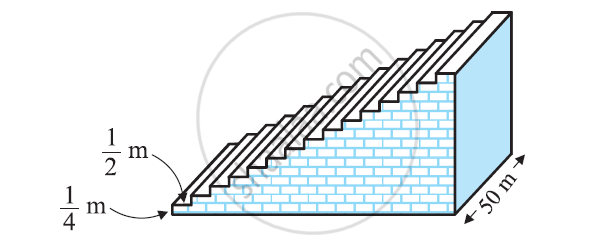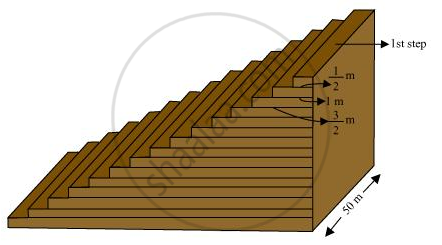Share

# A small terrace at a football ground comprises of 15 steps each of which is 50 m long and built of solid concrete. Each step has a rise of 1/4 m and a tread of 1/2 m (See figure) calculate the total volume of concrete required to build the terrace. - CBSE Class 10 - Mathematics

ConceptSum of First n Terms of an AP

#### Question

A small terrace at a football ground comprises of 15 steps each of which is 50 m long and built of solid concrete. Each step has a rise of 1/4 m and a tread of 1/2 m (See figure) calculate the total volume of concrete required to build the terrace.[Hint : Volume of concrete required to build the first step = 1/4 x 1/2 x 50m3]

#### SolutionFrom the figure, it can be observed that

1st step is 1/2 m wide,

2nd step is 1 m wide,

3rd step is 3/2 m wide.

Therefore, the width of each step is increasing by 1/2 m each time whereas their height 1/4 m and length

50 m remains the same.

Therefore, the widths of these steps are

1/2,1, 3/2, 2,...

Volume of concrete in 1st step = 1/4 xx1/4 xx50 = 25/4

Volume of concrete in 2nd step = 1/4 xx 1xx 50 = 25/2

Volume of concrete in 3rd step = 1/4 xx 3/2 xx 50 = 75/4

It can be observed that the volumes of concrete in these steps are in an A.P.

25/4,25/2, 75/4,...

a = 25/4

d = 25/2 - 25/4 = 25/4

and

S_n = n/2[2a + (n - 1)d]

S_15 = 15/2[2(25/4)+(15-1)25/4]

=15/2[25/2+((14)25)/4]

= 15/2[25/2 + 175/2]

=15/2(100) = 750

Volume of concrete required to build the terrace is 750 m3.

Is there an error in this question or solution?

#### APPEARS IN

NCERT Solution for Mathematics Textbook for Class 10 (2019 to Current)
Chapter 5: Arithmetic Progressions
Ex. 5.40 | Q: 5 | Page no. 115

#### Video TutorialsVIEW ALL 

Solution A small terrace at a football ground comprises of 15 steps each of which is 50 m long and built of solid concrete. Each step has a rise of 1/4 m and a tread of 1/2 m (See figure) calculate the total volume of concrete required to build the terrace. Concept: Sum of First n Terms of an AP.
S